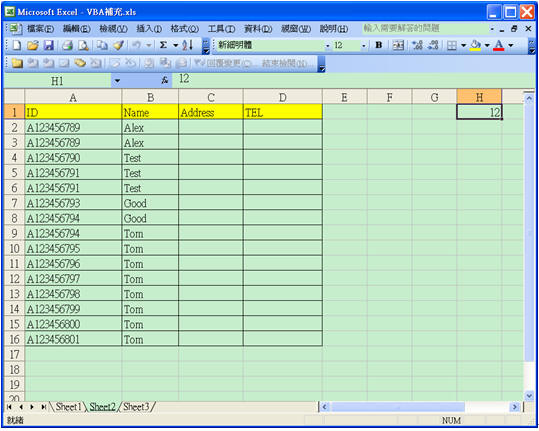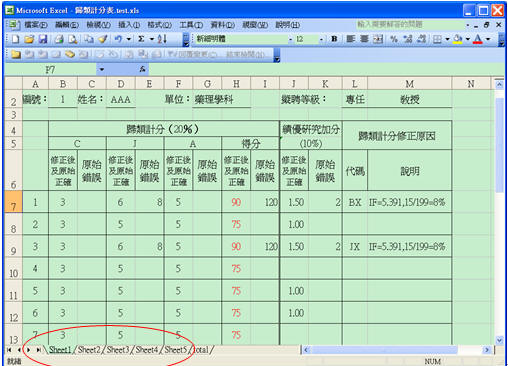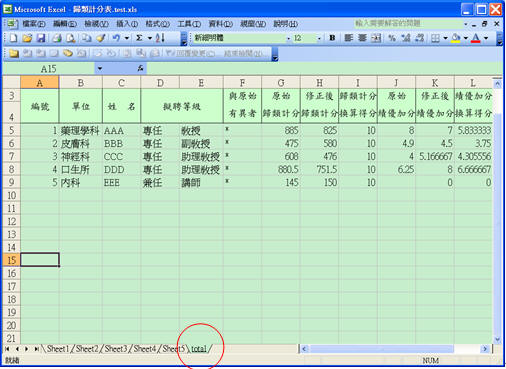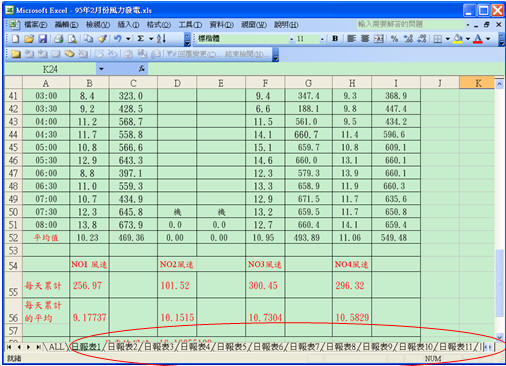﻿ EXCEL巨集實用範例

# ntuccepaper2019

## 技術論壇

### 手機版選單

#### 字級

EXCEL巨集實用範例
• 卷期：v0019
• 出版日期：2011-12-20

### 第一階段：以(Range("A" & I)) 模擬一維陣列Sub countunique()

Dim I, count As Integer

I = 2  '第一列是標題 資料從第二列開始

count = 0  '計數器變數

Worksheets(2).Select  '資料放在Sheet2

'將第一筆資料的ID放到AA變數以便和下一筆比較

AA = Range("A" & I).Value

I = I + 1  '指向下一筆資料

Do While AA <> ""  '還有記錄資料 即ID不等於空字串

'假使ID值不等於AA變數的上一筆ID值 則計數加一

If Range("A" & I).Value <> AA Then

count = count + 1      '不重複的ID則計數器變數加一

'將目前的ID保留到變數AA以便和下一筆ID比較

AA = Range("A" & I).Value

End If

I = I + 1  '指向下一筆資料

Loop

'迴圈結束表示不再有ID資料了 把加總計數結果放到H1儲存格

Range("H1").Value = count

End Sub

Sub delsame()

Dim I As Integer

I = 2

Worksheets(2).Select  '資料放在Sheet2

'將目前的ID和Name保留到變數AA和BB以便和下一筆比較

AA = Range("A" & I).Value

BB = Range("B" & I).Value

I = I + 1 '指向下一筆資料

Do While AA <> ""  '還有記錄資料 即ID不等於空字串

'假使ID等於上一筆ID , Name等於上一筆Name則該筆刪除

If Range("A" & I).Value = AA And Range("B" & I).Value = BB Then

Rows(I).Delete

Else

'不然繼續將目前的ID和NAME保留到變數AA和BB以便和下一筆比較

AA = Range("A" & I).Value

BB = Range("B" & I).Value

I = I + 1 '指向下一筆資料

End If

Loop

End Sub

### 第二階段：以(Cells(I, J)) 模擬二維陣列Sub Array_Mult()

Dim I, J, K, temp As Integer

Worksheets(1).Select

For K = 1 To 3

For I = 1 To 3

temp= 0

For J = 1 To 2

temp = temp + (Cells(I + 2, J + 1) * Cells(J + 2, K+ 4))

Next J

Cells(I + 6 , K + 1) = temp

Next I

Next K

End Sub

sheet1:原始明細檢驗資料。sheet2:合併後一個病歷號一筆資料。Sub Rearrange()

Dim I, J, M, N As Integer

I = 2    '表示原始明細資料的第幾列

M = 1  '表示合併後資料的第幾列

N = 1  '表示合併後資料的第幾欄

Worksheets(1).Select  '選擇原始明細檢驗資料

oldID = ""  '用來保留前一筆的病歷號

ID = Range("A" & I).Value '將目前的病歷號保留到變數ID以便和下一筆比較

Item = Range("B" & I).Value '將目前的檢驗項目保留到變數Item以便比較

Value1 = Range("C" & I).Value '將目前的檢驗值保留到變數Value1以便比較

Do While ID <> ""  '還有記錄資料 即ID不等於空字串

Worksheets(2).Select  '選擇合併後一人一筆資料

N = 1 '合併資料從第一欄開始比對檢驗項目

If ID = oldID Then '同一病歷號則開始合併

Do While Item <> Cells(1, N) '檢查檢驗項目是否相同

N = N + 1 '檢驗項目不同則繼續找下一欄位

Loop

Cells(M, N) = Value1  '檢驗項目相同則在此位置放入檢驗值

Else '當開始一個新的病歷號時

M = M + 1  '合併檔往下新增一列

Cells(M, N) = ID '加入新的一筆病歷號

Do While Item <> Cells(1, N) '檢查檢驗項目是否相同

N = N + 1 '檢驗項目不同則繼續找下一欄位

Loop

Cells(M, N) = Value1 '檢驗項目相同則在此位置放入檢驗值

End If

Worksheets(1).Select      '再切回原始明細檢驗資料

oldID = ID       '將病歷號保留以便和下一筆比較

I = I + 1    '移到下一筆

ID = Range("A" & I).Value '將目前的病歷號保留以便和下一筆比較

Item = Range("B" & I).Value '將目前的檢驗項目保留以便和下一筆比較

Value1 = Range("C" & I).Value '將目前的檢驗值保留以便和下一筆比較

Loop

End Sub

### 第三階段：以Worksheets("sheet" & N)模擬三維陣列Sub TOTAL()

Dim i, j As Integer

Dim sh As String

i = 5  ' total sheet 從第五列開始

For N = 1 To 5  '總共有sheet1-sheet5

sh = "sheet" & N  ' 工作表名稱

Worksheets(sh).Select  '選擇某頁工作表

Value1 = Range("G2").Value '需彙總的儲存格值分別放入各個變數

Value2 = Range("D2").Value '需彙總的儲存格值分別放入各個變數

Value3 = Range("L2").Value '需彙總的儲存格值分別放入各個變數

……

Worksheets("TOTAL").Select  '選擇TOTAL  sheet

Range("A" & i).Value = N '放入這是第幾位的編號

Range("B" & i).Value = Value1 '將各個變數值分別放入儲存格位置

Range("C" & i).Value = Value2 '將各個變數值分別放入儲存格位置

Range("D" & i).Value = Value3 '將各個變數值分別放入儲存格位置

……

i = i + 1 '彙總頁total往下新增一列 5頁明細表就有5列資料

Next

End Sub